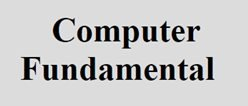# Number System with its Types and Conversion

## Definition of Number System

Number system defines a set of value used to represent quantity.

Number system is a language of digital system consisting of a set of symbol called digits with rules defined for addition, multiplication and other mathematical operation.

Base or radix of a number system is the number of symbol used in its representation.

## Types of Number System

There are four types of number system in general:

1. Decimal number system
2. Binary number system
3. Octal number system

## Bases of all Number System

There are various types of number system available as given in the following table with its base/radix:

Number System Base
Binary 2
Octal 8
Decimal 10

## Symbols used in Number System

The decimal number system uses 10 symbols, that are 0, 1, 2, 3, 4, 5, 6, 7, 8, 9.

The binary number system uses only 2 symbols, that are 0 and 1.

The octal number system uses 8 symbols, that are 0, 1, 2, 3, 4, 5, 6, 7.

The hexadecimal number system uses 16 symbols, that are 0, 1, 2, 3, 4, 5, 6, 7, 8, 9, A, B, C, D, E, F.

## Decimal Number System

The decimal number system is also called as the base-10 system because it has total of 10 digits to use, that are from 0 to 9.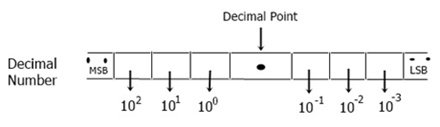## Binary Number System

Binary number system is the most important and valuable number system from all the number system for computer point of view, as we all knows that a computer can not operate on the decimal or any other number system because it only operate using binary logic.

This number system only uses 2 digits or symbols, that is 0 and 1. Or we can says that this system will only depend on 0 and 1, or operate on 0 and 1 basis.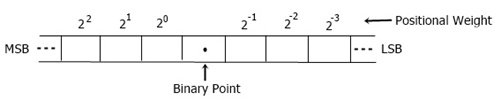## Octal Number System

Octal number system uses digits from 0 to 7, that is total of 8 digits or symbols.This number system uses 16 digits or symbols. In 16 digits, 10 digits are from 0 to 9 and other 6 are from A to F.## Number System Conversion

Now we will talk about conversion from one number system to another number system using some method discussed here.

Here are the list of conversion that are going to be discussed in this article or tutorial.

• binary to decimal
• binary to octal
• decimal to binary
• decimal to octal
• octal to decimal
• octal to binary

Now let’s learn about method to apply all the conversion as given or listed in the above list.

### Binary to Decimal Conversion

To convert any number given in binary form or binary number system into a decimal number system, then coefficient of binary number are multiplied by the power of 2. Let’s take an example.

Convert (110110)2 in binary form.

(110110)2 = ( ? )10
= 1*25 + 1*24 + 0*23 + 1*22 + 1*21 + 0*20
= 32 + 16 + 0 + 4 + 2
= ( 54 )10

Therefore, 110110 in binary will be equal to 54 in decimal.

### Binary to Octal Conversion

We have two method or way to convert any binary number to octal.

#### First Way

In first way of converting binary to octal, we have to convert the given binary number to decimal number and then convert the decimal number to the octal number.

#### Second Way

In second way for converting binary to octal, we have to follow all the steps given below:

1. Group the binary bit into group of 3-3 parts, starting from LSB
2. Place 1 or 2 zero as required before binary numbers, in case if the total number of digits is not divisible by 3. As we have to make pairs of 3-3 digits, then what if we have 8 digits like 10110100, 8 is not divisible by 3, then we have to append 0 before 10110100 to make it 010110100.
3. Now convert each group as its equivalent decimal.

Now let’s take an example of how to convert the number 11010010 in binary form, to octal number system.

Or, convert (11010010)2 = (?)8.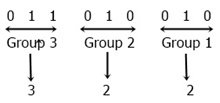As you can see from the above figure, after converting the number 11010010 given in binary form into octal number system, we get 322.

Therefore, (11010010)2 = (322)8

To convert any number from binary to hexadecimal number system, we have to follow the given steps:

1. Split the digits of binary number into 4-4 pairs.
2. Start from LSB to the MSB for making pair of 4-4 bits or numbers.
3. Now convert each 4-bit binary number to its equivalent hexadecimal number.
4. Now combine all the converted number, that will be the same number, but in hexadecimal number system.

Let’s take an example to see how we can convert a binary number to hexadecimal number.

We have a number say 1010111110110010, now we have to convert it into hexadecimal form. See the figure given below: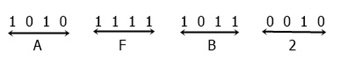As you can see from the above figure, we have got total of 4 digits that is A, F, B, and 2. Now combine all the four digit, we will get AFB2 that is the same number, but in hexadecimal form.

Therefore, (1010111110110010)2 = (AFB2)16

### Decimal to Binary Conversion

To convert any decimal number to binary number, we have to divide the decimal number by the base of binary number system which is 2. Let’s take an example for better understanding about the working of this conversion.

We have a number say 105 given in decimal number system, now we have to find the same number or equivalent number in binary form or binary number system. Let’s concentrate on the figure given below: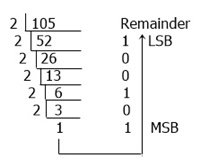From the above figure, we will get 1101001, which is the same number as 105, but in binary number system.

Therefore, (105)10 = (1101001)2

Now let’s learn about conversion of fractional part from decimal to binary.

Here are the steps or rules of multiplication for fractional part conversion:

• Multiply the given fractional decimal number by the base r (2 here)
• Now record the carry generated in this multiplication as HSB
• Multiply only the fractional number of the product in step 2 by the base 2, and record the carry as the next bit to MSB.

Let’s take an example to apply the above steps practically and learn how we can convert any fractional part value given in decimal number system to binary version of the same fractional part.

Here in the example, we have to convert .42 to binary version. That is, (.42)10 = ( ? )2.

The table given below shows the step by step multiplication of the fractional part as directed in the above rules.

Decimal Fractional Multiply with Base Multiplication Result Carry Value
.42 * 2 0.84 0 MSB
.84 * 2 1.68 1
.68 * 2 1.36 1
.36 * 2 0.72 0
.72 * 2 1.44 1 LSB

As you can see the multiplication procedure, first we have multiply the complete fractional part, that is .42 with base, that is 2. Then we have got the value 0.84 as product, and in this number we have carry record as 0, as we have to take the number present before decimal point, therefore 0 is the carry record here. And after multiplying .84 with 2, then we have got 1.68 as multiplication result, and here we can see that the carry is 1. Similarly, in third, fourth and fifth multiplication, we have carry as 1, 0 and 1.

Now after combining all the carry record, we will get 01101 as result. Therefore, this is equivalent to the .42, which is 0.01101.

Therefore, (0.42)10 = (0.01101)2.

### Decimal to Octal Conversion

Now let’s learn about how we can convert any number given in decimal number system to octal number system.

Here, we have to divide the decimal number by the base of the octal number system or 8. Let’s take an example to understand it in a clear way.

(204)10 = ( ? )8.

As you can see from the above example, we have to convert the number 204 to octal number system.Look at the above figure carefully. Here we have first divided the number 204 with 8, we have got 25 as result and 4 as remainder, now again divide 25 with 8. Continue dividing until the number becomes less than that number through with you are dividing, that is 8 here.

Always write the remainder at the right side of the result. After dividing the number or after getting the remainder less than 8, stop dividing and write the remainder from last to first. Here we have, 314 as result or octal value of the same number given in decimal number, that is 204.

Now let’s learn about how to convert any decimal number to the equivalent number in hexadecimal system.

Here in hexadecimal number system, the base is 16, therefore we have to divide the decimal number with 16 and follow the same rule as learned in conversion of decimal to octal.

Let’s take an example to understand this conversion method clearly.

(259)10 = ( ? )16From the above figure, we have got the value 103 in hexadecimal system, that is the same number.

Hence, (259)10 = (103)16

### Octal to Binary Conversion

To convert any octal number into binary, we have to convert each and every octal digit into the 3-bit binary number. Let’s take an example.

Convert the octal number, 364 to binary system. Or (364)8 = ( ? )2.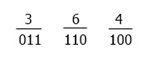From the above figure, we have 3 3-bit binary number, the first one is 011, the second one is 110, and the third one is 100. Therefore on combining, we have 011110100.

And the result is, (364)8 = (011110100)2.

### Octal to Decimal Conversion

We have to follow these rules given below to convert any octal number to decimal system.

1. Write down the given octal number
2. Write down the weight for different position
3. Multiply each digit from the given octal number with the corresponding weight to obtain the produce
4. Now add all the product value to get the decimal equivalent of the given octal number.

Now let’s take an example and apply all the above rules practically to understand it in a better and clear way.

For example, we have to convert the number 315 given in octal system into decimal system.

Or simply, (315)8 = ( ? )10

Now concentrate on the table given below, that shows how to apply all the above listed rules to convert octal to decimal.

 3 1 5 Given Number 82 81 80 Weight

Now apply the rules number 4 to get the result, that is

= 3*82 + 1*81 + 5*80

= 192 + 8 + 5

205 is the answer, or the decimal equivalent.

Therefore, (315)8 = (205)10

To convert any octal number to hexadecimal system, we have to first convert that octal number into binary, and then convert that binary number into hexadecimal number.

For example, (435)8 = ( ? )16

We have to convert the above number, 435 given in octal system into hexadecimal number system. Then we have to convert its binary equivalent. The figure given below shows its binary equivalent or binary equivalent of 435.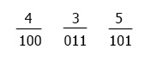According to the figure given above, we have 100 011 101 is the value, that is binary equivalent of octal number 435. Now let’s convert it into hexadecimal system. Or we have to calculate, (100011010)2 = ( ? )16

Before proceeding, we have to make 4-4 pair of binary bit, and here we have total of 9 digit, therefore to make 4-4- pair, we have to add 3 zero before the number, after adding three zero we have 000100011101. Now make 4-4 pair and convert each pair into it’s hexadecimal equivalent as shown in the figure given below.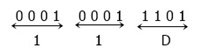According to the above figure, we have hexadecimal equivalent is 11D. Therefore, (435)8 = (11D)16

To convert any hexadecimal number to its binary equivalent, we must have to follow these rules:

1. Convert each hex digit to its 4-bit binary equivalent
2. Combine all the 4-bit binary equivalent

Let’ s take an example to understand and apply the above rules.

(AFB2)16 = ( ? )2

Here we have to convert the number AFB2 given in hexadecimal to binary system. Let’s take a look at the table given below, that shows you the conversion of the above hexadecimal number to its binary equivalent.

Hex Digit 4-bit Binary Equivalent
A 1010
F 1111
B 1011
2 0010

From the above figure, we have 4 4-bit binary equivalent, the first one is 1010, the second one is 1111, the third one is 1011, and the fourth one is 0010. On combining all the four 4-bit binary number, we get 1010111110110010. Therefore, (AFB2)16 = (1010111110110010)2

To convert any hexadecimal number into decimal system, we have to follow the rules given below:

1. Write down the given hexadecimal number
2. Write down the weight for different position
3. Now multiply each and every digit from the given hexadecimal number with the corresponding weight to obtain the product
4. Now add all the product to get the decimal equivalent<

Now let’s take an example and apply the rules given above to understand it in a clear way.

We have a number say 1D7F in hexadecimal system, now we have to convert it into decimal equivalent.

That is, (1D7F)16 = ( ? )10

The table given below shows you how to apply first 3 rule as given above, to convert hexadecimal to decimal:

 1 D or 13 7 F or 15 Given Number 163 162 161 160 Weight

After concentrating on the above table, now apply rule number 3 and 4, then we have

= 1*163 + 13*162 + 7*161 + 15*160

= 4076 + 3328 + 112 + 15

= 7551, which is the decimal equivalent of the given hexadecimal number, that is 1D7F.

Therefore, (1D7F)16 = (7551)10

To convert any hexadecimal number to octal number system, we have to follow all these rules:

1. Write down 4-bit binary number from each and every hex digit
2. Combine these 4-bit binary numbers
3. Now split the above binary number into 3 3-bit pair starting from LSB side
4. Now convert each of the above 3-bit group into its equivalent octal digit

Let’s take an example to apply all the above rules and understand it in a clear way of converting hexadecimal number to octal system.

(4CA)16 = ( ? )8

Therefore we have a number in hexadecimal system that is, 4CA. We have to split that number and convert it into a 4-bit binary equivalent as shown in the table given below:

Hex Digit 4-bit Binary Equivalent
4 0100
C 1100
A 1010

Therefore, we have total of 3 pair of 4-bit binary numbers, that is 0100, 1100, and 1010. Now combine all the 3 pair, we get 010011001010. Now we have to make or split it into 3-3 pair. Then we get, 010, 011, 001, and 010. Convert these 4 pair of 3-bit binary number into its equivalent octal value as shown in the figure given below:From the above figure, on combining all the 4 octal equivalent, we get 2312.

Therefore, finally (4CA)16 = (2312)8

Tools
Calculator